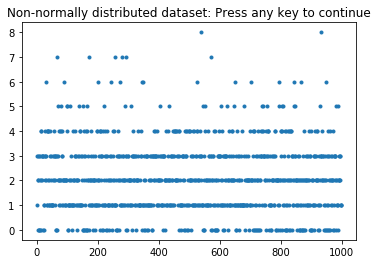# Bootstrapping¶

Example of bootstrapping the confidence interval for the mean of a sample distribution Since no bootstrapping is implemented in Python, this function requires "bootstrap.py", which is included.

Note: The original scikits-bootstrapping module, which works only under Python 2, is available from http://github.org/cgevans/scikits-bootstrap

Author: Thomas Haslwanter, Date: Feb-2017

In :
%matplotlib inline
import scipy as sp
import matplotlib.pyplot as plt
from scipy import stats
import scikits.bootstrap as bootstrap

In :
# Generate a non-normally distributed datasample
data = stats.poisson.rvs(2, size=1000)

# Show the data
plt.plot(data, '.')
plt.title('Non-normally distributed dataset: Press any key to continue')

Out:
<matplotlib.text.Text at 0x27e6c3619e8>In :
# Calculate the bootstrap
CIs = bootstrap.ci(data=data, statfunction=sp.mean)

# Print the data: the "*" turns the array CIs into a list
print('The conficence intervals for the mean are: {0} - {1}'.format(*CIs))

The conficence intervals for the mean are: 2.001 - 2.179